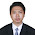## Friday, May 1, 2009

### Spherical geometry optimisationsI am currently working on recoding proper classes for managing spherical geometry primitives in Stellarium. One of the most used primitive is the so called spherical cap showed in the figure. A spherical cap (sometimes called half space) is a disk-like region of the sphere defined by a direction unit vector n and an aperture angle ⍺.

The most common operation that we want to perform on a spherical cap is to check if a point A of the sphere lies inside the cap. This is pretty easy to do by checking whether the dot product n·OA is smaller than cos(⍺).

The C++ code below implements this. Note that to avoid computing a CPU expensive cosine each time we perform the contains operation, we pre-compute cos(⍺) and store it as a class member d.

class SphericalCap
{
bool contains(const Vec3d &v) const {return (v*n>=d);}
Vec3d n;
double d;
};

Another useful operation we want to perform often is to check whether two spherical caps with direction vectors n1 and n2 and aperture ⍺1 and ⍺2 intersect. The straightforward implementation is to compute the angle θ between n1 and n2 and check whether it is smaller than ⍺1 + ⍺2.

bool intersects(const SphericalCap& other) const
{
const double theta = n.angle(other.n);
return theta < acos(d) + acos(other.d);
}

Unfortunately, because we store only the cosine of ⍺1 and ⍺2, this solution involves 2 calls to the acos function, plus 1 call to the angle() function which itself involves 1 acos and 1 sqrt. Needless to say that it's not lightning fast..

After some thoughts I finally came up with a solution involving only basic arithmetics:

First, in the case ⍺1+⍺2 >= 180°, (i.e. if d1+d2<=0) the 2 caps necessarily intersect so we don't need to think further. Let's now treat the other cases:

The 2 caps intersect only and only if:

θ <= ⍺1+⍺2         (1)

Because both members of (1) are < 180° (the angle θ between n1 and n2 can obviously not be > 180°), we can transform (1) into:

cos (θ) >= cos(⍺1+⍺2)

which with basic trigo can be transformed into:

L <= sin ⍺1 sin ⍺2 , with L = cos ⍺1 cos ⍺2 - cos θ     (2)

In our case L is known and given by d1*d2-n1·n2. Because ⍺1 and ⍺2 are bounded between 0° and 180°, sin ⍺1 sin ⍺2 is bounded between 0 and 1. Therefore if L<=0, (2) is verified and the 2 caps intersect. Similarily, if L>1, (2) is not verified and the 2 caps don't intersect. In the last case where 0<L<=1 we can transform (2) into:

L² <= sin²⍺1 sin²⍺2

which with basic trigo formulae can be transformed into:

L² <= (1-cos²⍺1)(1-cos²⍺2)         (3)

In our case cos²⍺1 and cos²⍺2 are know and given by d1² and d2² so we can easily check that (3) is verified or not.

We thus have a solution to each cases, and none of them involve any CPU expensive operation!! In C++ code, this reasoning translates into:

bool intersects(const SphericalCap& other) const
{
const double L = d*other.d - n*other.n;
return d+other.d<=0. || L<=0. || (L<=1. && L*L <= (1.-d*d)*(1.-other.d*other.d));
}

#### 1 comment:

1.excuse me,
i'm a student college, i'm come from indonesian and i want ask something about stellarium because i'm beginner in here. i've a problem in my college, i've got a final project. it's about stellarium my final project title is "SIMULATOR SATELITE" and i've got a task to build a project using stellarium, in this case i have to control a satelite in stellarium using ethernet, where the satelite can be controlled using another PC. each PC can be comunication each other using a protocol in ethernet, in this case i using UDP to transfer data. so we can controlled a satelite in stellarium using another PC. that PC can send data about controlled the satellite using another PC. i've read documentation about stellarium, it's built using C++, and my final project using C++ too. in this case i'm using socket programing in C++ but i've got many problem within process of finishing. i don't know how first i have to do? so i want ask any some question?
1. Do you have a solution to guide me, please sir...
2. could we send data to controlled a satelite or orbit using another PC, in this case using Socket Programming C++/C within a network or ethernet, so i can controlled a satellite or orbit using another PC?

thank you